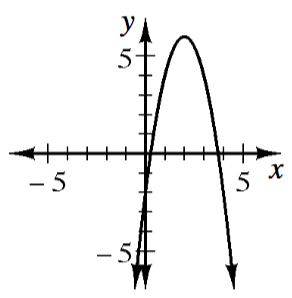### Home > INT2 > Chapter 9 > Lesson 9.3.1 > Problem9-82

9-82.

Consider the quadratic function $f(x) = -2(x - 2)^2 + 6$.

1. Without drawing a graph, determine the coordinates of the vertex and state whether the vertex represents the maximum or minimum value of the function.

The vertex is the point where $-2(x - 2)^2 = 0$

The vertex is a maximum when the parabola opens downward and a minimum when the parabola opens upwards.